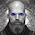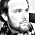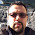Friday, 26 April 2013

Calculate RMSD from two XYZ files

I want to calculate the RMSD (Root-mean-square deviation) between two molecule structures in XYZ format. And after googling around I concluded that the easiest way to do it was to use pymol. However being a CLI user, I do not want to download the files and open up a GUI all the time, I just want a script that can do it via a terminal. Time for a little google and python project.

Calculating the RMSD mathematically for two sets of xyz coordinates for $n$ particles is straight forward:

$$\mathrm{RMSD}(v,w) = \sqrt{ \frac{1}{n} \sum_{i=1}^n ((v_{ix} - w_{ix})^2 + (v_{iy} - w_{iy})^2 + (v_{iz} - w_{iz})^2 ) }$$

However this is without taking into account that the two molecules could be identical and only translated in space. To solve this we need to position the molecules in the same center and rotate one onto the other.

The problem is solved by first find the centroid for both molecules and translating both molecules to the center of the coordinate system. Then we need an algorithm to align the molecules by rotation. For this I found Kabsch algorithm from 1976.

"It is a method for calculating the optimal rotation matrix, that minimzes the RMSD between two paired set of points.http://en.wikipedia.org/wiki/Kabsch_algorithm

The algorithm is nicely written out on wikipedia, so it was straight forward to implement (still took me a little time though). So I wont go into details of it here.

However it is clear that the centering the molecules using their centeroid could possibly not be the best way of finding the minimal RMSD between two vector sets. So +Lars Bratholm got the idea of using a fitting function, fitting the center of the molecules and using Kabsch to calculate a minimal RMSD.

Code:
You can find the code and examples here: github.com/charnley/rmsd

Usage:


./calculate_rmsd.py molecule1.xyz molecule2.xyz


Results:
The output will then be "pure rmsd", "rmsd after rotation" and "rmsd after fit".

Note; for a testcase I calculated the rmsd for a molecule set with 140 atoms, to be ~0.15, and when I did the same calculation in pymol I got 0.092. However pymol did state that it was cutting 20 atoms, but didn't state which, where as my script takes all the atoms into account.

Happy RMSD'ing everyone.

1.You should really use OpenBabel to read files! Why restrict your script to XYZ files? :)

2.I thought about it, but I wanted the script to be as simple as possible, and XYZ is a very simple format. It is not everyone who has OpenBabel with Python bindings on their computer.

3.Tinker uses an interesting quaternion based method.

http://dasher.wustl.edu/tinker/distribution/source/quatfit.f

1.The quaternion based method was also mentioned on wikipedia, but I found it a bit harder to implement at the time. The fortran you routine you linked too seem pretty readable though. Thx.

2.4.Sry for digging up an old post:
"Using quaternions to calculate RMSD"
http://onlinelibrary.wiley.com/doi/10.1002/jcc.20110/abstract

The fortran code can be found online as well.
It expects PDBs, but writing a quick subroutine for xyz-files is quickly done

5.Hi, I found very useful your algorithm, I would like cite your work. Have a nice day!

1.hi, I'm glad you found it useful. Please find citation notes on the github page https://github.com/charnley/rmsd

6.Is there a way to compare more than 2 .xyz files? I have multiple calculations of similar structures and I'm thinking I have about 8 files I would like to compare. Is it possible for the script to handle all 8 at once? Thanks

1.Hi Sean, Sorry, it is only possible to calculate RMSD between two xyz files.

2.That's cool. I have to work out the clock anyway. :)

7.Hi I have an MD trajectory and want to work out the RMSD over that trajectory for each bond in the molecule (single amino acid) individually. The trajectory file gives me ~ 7000 snapshots i.e. 7000 xyz files is there a way to achieve this by loop your code or is your method generalisable to work only for selected atoms in a structure?

1.Hi, I don't think you need this algorithm for that. You can just define a list of atom pairs and calculate the actual distance between those pairs. No need for any translation.

2.Sorry for the double post I realised it had posted anonymously the first time. Thanks for your response, I'll give that a go.

8.Hi I have an MD trajectory and want to work out the RMSD over that trajectory for each bond in the molecule (single amino acid) individually. The trajectory file gives me ~ 7000 snapshots i.e. 7000 xyz files is there a way to achieve this by loop your code or is your method generalisable to work only for selected atoms in a structure?

9.I don't want to self promote, but I just created a repository with a script that does clustering of structures of a trajectory (with trajectory meaning a set of configurations of the same molecule in the same file).
It uses OpenBabel to read the configurations (as suggested in this comments section) and do a clustering analysis with SciPy.
The distances are based on Jimmy's impĺementation of RMSD with the Kabsch algorithm (and uses the rmsd library).

I think this might be useful for some people (it was for me) so you might want to check it out
https://github.com/hmcezar/clustering-traj

1.Very cool.

10.11.What's the solution for molecules of different sizes?

12.